Data Science

# Unit Testing of Machine Learning with Test Driven

Jagreet Kaur | 31 January 2022

### Table of Content

SubscriptionThanks for submitting the form.## Machine Learning Unit Testing with Test-Driven Development

A pattern built for development in performance testing is known as Test-Driven Machine Learning Development. It is a process that enables the developers to write code and estimate the intended behavior of the application. The requirements for the Test-Driven Machine Learning Development process are mentioned below-
• Detect the change in intended behavior.
• A rapid iteration cycle that produces working software after each iteration.
• To identify the bugs. If a test is not failing, but still a bug is found, then it is not considered as a bug, it will be considered as a new feature.
Tests can be written for functions and methods, whole classes, programs, web services, whole machine learning pipelines, neural networks, random forests, mathematical implementations, and many more.
You May also Love to Read Overview of Artificial Neural Networks and its Applications

## Test-Driven Machine Learning Development Lifecycle

The TDD cycle enables the programmer to write functions in small modules. The small test modules consist of three sections that are described below -
• Failed Test (RED) - The First step of Test-Driven Development is to make a failure test of the application. In terms of Machine Learning, a failure test might be the output of an algorithm that always predicts the same thing. It is a kind of baseline test for Machine Learning algorithms.
• Pass the Failed Test (GREEN) - After writing the failed test, the next move is to pass the written failed test. The failed test is divided into a number of small failed tests and then tested by passing random values and dummy objects.
• Refactoring the Code - After passing the failed test, there is a need to refactor the code. To implement the refactoring process, one must keep in mind that while making changes in the code the behavior should not be affected.
If the developer is adding a special handling feature in the code such as an if statement, the code will no longer follow the refracting process. If while refactoring the code, the previous test alters then the code has to pass the test process cycle.

## What is Acceptance Test-Driven Development?

ATDD stands for Acceptance Test-Driven Development. This technique is done before starting the development and includes customers, testers, and developers into the loop. These all together decided acceptance criteria and work accordingly to meet the requirements. ATDD helps to ensure that all project members understand what needs to be done and implemented. The failing tests provide us quick feedback that the requirement is not being met.

### Advantages of Acceptance Test-Driven Development

• As we have ATDD very first, so it helps to reduce defect and bug fixing effort as the project progresses.
• ATDD only focuses on ‘What’ and not ‘How’. So, this makes it very easier to meet customers’ requirements.
• ATDD makes developers, testers, and customers work together, this helps to understand what is required from the system

Test Driven Development (TDD) is a programming practice which combines Test First Development (TFD) with refactoring. Click to explore about, Javascript and ReactJS Unit Testing, TDD and BDD

## Importance of ML Unit Testing and Code Coverage?

Many times, the code doesn’t raise an error. However, the result of the answers won’t be as expected or the other way around the output we get is not exactly what we wanted. Let us assume that we want to use a package and we start to import the same. There is a chance that the imported package must have already been imported and we are importing it again. Therefore, to avoid such a situation and we want to test if the package we wanted to import is already imported or not. So, when we submit the whole code to the test case, the test case should be able to find if the package is already imported or not. This is to avoid duplication. Similarly as above, when we wanted to use the pre-trained models for predictions, the models sometimes will be huge and we want to load the model only once and in the process, if we load multiple times, the processing speed gets slowed down due to occupying more memory which actually is not required.

Even in this case duplication has to be avoided. Other cases that we could look at are the sufficient conditions. If we create a function, the function will take in an input and returns an output. So, when we use the concept of necessary and sufficient conditions, we’re interested in knowing the sufficient condition to say that the function is working properly. To give an example of a necessary condition, each step in the function should be error-free. If we create a function and on giving the input if it raises error forex: indentation error, the function is not well defined. So, one of the necessary conditions is error-free steps. But, if the function runs successfully and gives an output, does that mean we have the correct answer?

Let’s say, we have two functions in a package, addition, and multiplication but the developer has actually given the code of addition for multiplication and vice-versa(a typo while defining the function). If we use the function directly we will get the result; we won’t get the expected results. So, we could create a test case where given any two known inputs and the known output, if not one, for a few test examples, we can set the condition saying if all the test cases pass, then the given function is correct.

### Machine Learning Unit Testing with Python

First of all, a simple testing module implemented in Python is described which is further used for TDD in Machine Learning and Deep Learning. To start writing the test, one has to first write the fail test. The simple failing test is described below - In the above example, a NumGues object is initiated. Before running the testing script, the script is saved by the name which is ended with _tests.py. Then move to the current directory and run the following command - nosetests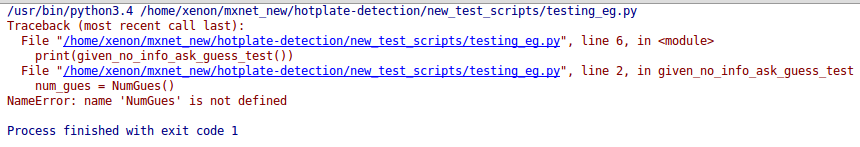By observing the above screenshot, it is concluded in the above code NumGues object is not defined. Therefore, a new class is made with NumGues.py where the class is developed. The code is mentioned below - class NumGues: "Guesses numbers based on the history of your input" After that import the class in the testing script by writing import NumGues at the top.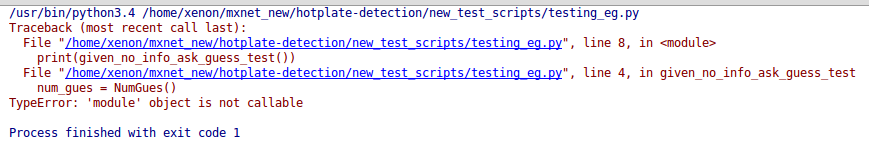The above screenshot says that the module object is not callable. Therefore, it is not the right way to call the class. Now call the class correctly at the top of the testing script and run it. from NumGues import NumGues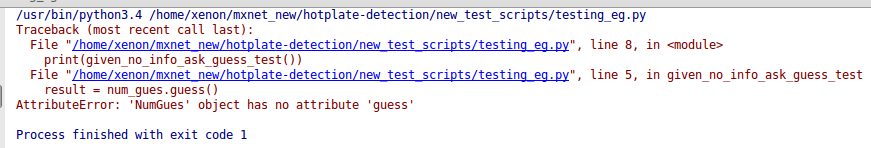From the above screenshot, it is concluded that the “guess” object is not present for the “NumGues” object. To remove the above error, some changes are made in the class. Changes are mentioned below - After that test results and observes the results from the below screenshots.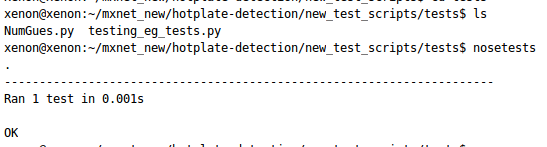## Test-Driven Machine Learning Process

TDD plays an important role in terms of machine learning. There are a number of issues that are faced while implementing machine learning algorithms. Therefore, with the use of TDD, it becomes easy to organize the code and solve issues efficiently. The issues while implementing machine learning is described below -
• Unstable Data - The Data Scientist generally removes outliers from the data to solve the issue of instability within the dataset. But, there may be a case in which instability doesn't get removed.
For example, if the input is considered in the range of 0 and 1 in binary format and the output is obtained in the range of -1 and 1 then, it will be a serious situation. This is because it can harm the applied model and even produces a hole within the time series.
• Underfitting - It is the situation when the model does not receive enough information to implement the algorithm accurately. This can be explained more with an example.
An exponential curve is developed using two points which can be taken as a linear relationship between the points. On the contrary, patterns are not obtained due to the presence of two points as a reference. If the range increases to view the results more clearly, the error also increases drastically. These situations are observed mostly in case of small datasets as the information available is not enough information that can be used to obtain an accurate result.
• Overfitting - When a smaller amount of data that is not ideal can be responsible for the occurrence of overfitting of the model. Overfitting occurs when the function effectively memorizes the data. But, the problem is that the function will not be responsible for the dataset outside the previous range of data. This is because it will work and train for a particular range of the dataset.
• Unpredictable Future - Machine Learning is taken as a powerful approach for predicting the future as the algorithms learn from the newly obtained information. But when the new information is received, some problems arrive or the data moves towards instability then, it becomes difficult to decide whether the model is working properly or not.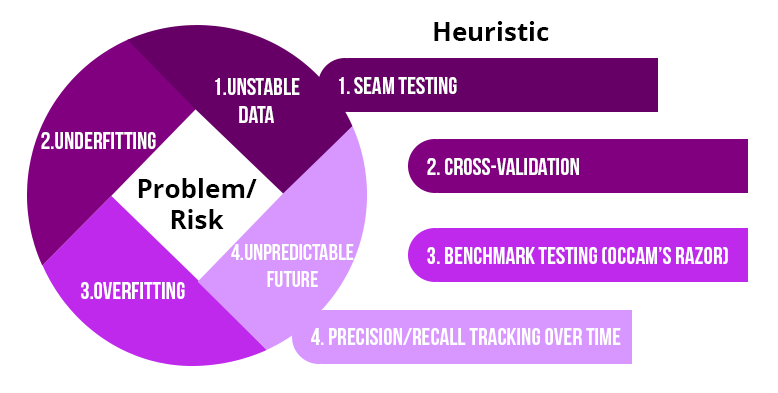### Test-Driven Machine learning Procedures to Reduce Risks

• Seam Testing - Machine Learning is not considered as legacy code, but it is similar to it. Therefore, machine learning algorithms can be considered as a black box.
Seam testing can be performed on the data flow of the machine learning algorithms. Therefore, unit testing is implemented on data inputs and outputs of the applied model to verify that the model is working within the given valid tolerances. Seam Testing is selected as it is one of the best approaches for testing the interfaces between the small modules of code.
• Examine the fitting of a model using Cross-Validation - In this case, the dataset is divided into two parts i.e. training and validation dataset.
• The first part (training set) is used to train the model. This is because machine learning works on the basis of mapping the previous observations to results. These algorithms learn from the historical data, therefore without learning, they cannot be used for required results.
• The test cases can be increased by splitting the dataset into smaller modules and perform the cross-validation process.
• The cross-validation process is implemented to reduce the real error rate whenever the new data is injected into the model.
• Reduce Overfitting by examining Speed of training - The complexity of the machine learning model is determined by the time they take to learn. For example, training the different models one takes 5 hours to train completely and the other takes just 40 min. Therefore, it can be concluded that the second model is better as compared with the first one.
An approach is implemented to monitor the speed of the model for training. The approach is known as the benchmark. It is one of the minutest of unit testing which is applied to the code to find when the learning speed of the model decreases or increases.
• Use of Precision and Recall for Monitoring Future Prediction - Precision and Recall are used for monitoring the implementation of machine learning algorithms. They are implemented using user input. They close the learning loop and feedback the information to improve the data more after observing the misclassification of the data.
Precision is responsible for monitoring the metrics of the percentage of true positives whereas Recall is defined as the ratio of true positives to true positives plus false negatives.
TDD is nothing but the development of tests before adding a feature in code. Click to explore about, Test and Behavior Driven Development and Unit Testing in Python

## What is a Developing Classifier?

A classifier can also be developed with the help of TDD. Each module of the classifier will be tested step by step to reach the final destination. Optimization of machine learning algorithms can be successfully implemented with the use of test-driven development (TDD). Working on the algorithm is then analyzed and the possibilities to improve it are evaluated with the help of TDD. The development of classifier can be explained clearly with the help of an example. Let’s say the algorithm works for the one-dimensional data. Therefore, optimization could be performed to enable the algorithm for multidimensional data. The test is described below that takes multidimensional data as input. After testing the above code the test is failed. The output is given below in the form of the screenshot -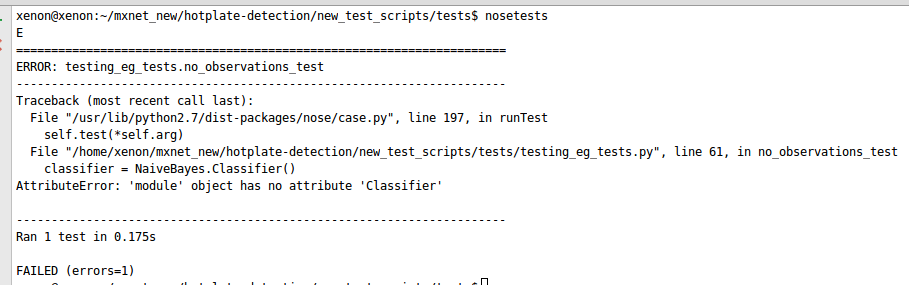From the above screenshot, it is observed there is a need to define “Classifier”. Therefore, class “Classifier” is made which is mentioned below - The above code depicts the class of Classifier which is used for performing the classification process. In order to work classification for single data, the code is given below:

## Testing ML Model with Test-Driven Development Approach

Testing of the working of a model is an important aspect in terms of the developer. Sometimes, there may be a situation that the code model is non-erroneous but the performance and accuracy of the model are worse. The reason behind this is difficult to find. Therefore, to accomplish this task TDD can be used, and working on a model could be improved efficiently. Let's take an example. Firstly, data is generated by performing logistic regression. The code is given below - Now, the next step is to develop the test for the model. The code for testing is mentioned below - The result of running the above code is given below -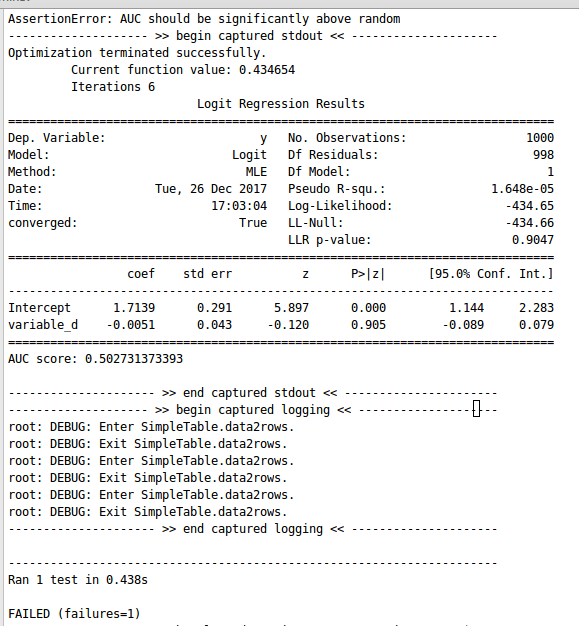After observing the above screenshot, it is concluded that the value of AUC is quite low i.e. 0.50. As well as the value of p-value is very much greater than 0.05. Therefore, it is quite understood that the model is worse. Now, the model can be tested for other variables as well. Let’s go for testing for variable_e and check the model accuracy. The results are displayed below -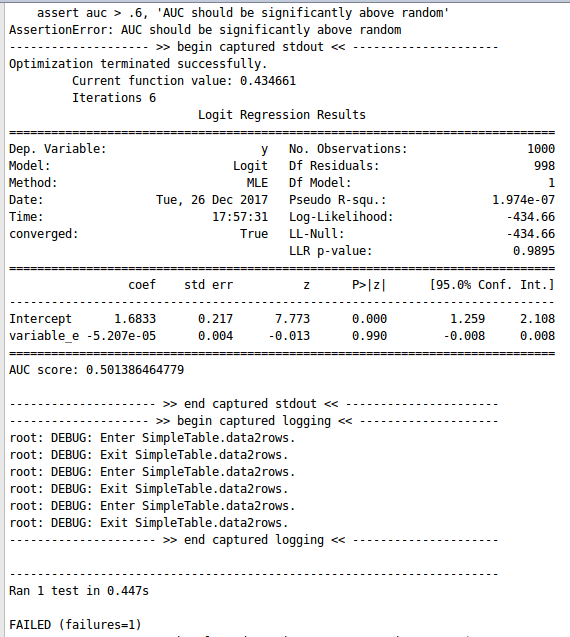After observing the above screenshot, it is concluded that still, the accuracy of the model is bad. Now, let’s try for variable_c. The results are shown below -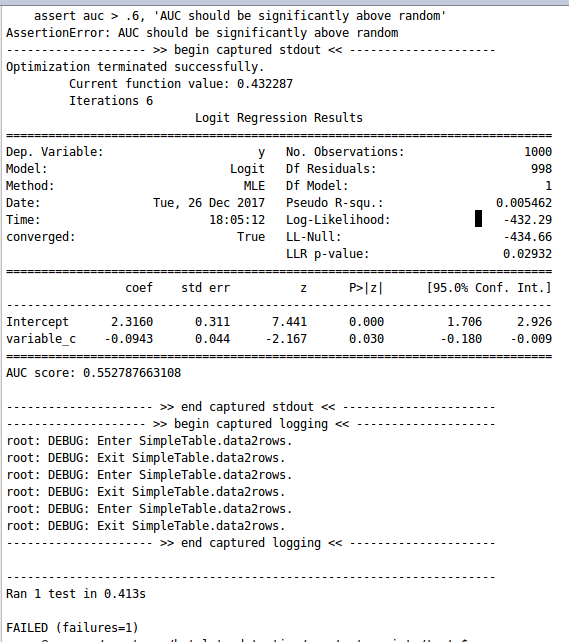From the above screenshot, it is concluded that the value of AUC is still low but the value of the p-score drastically decreases to 0.030. Therefore, the accuracy of the model is improving. Let’s increase the variables and test for both variable_b and variable_c. After implementation, it is observed that the test is passed. The screenshot is shown below -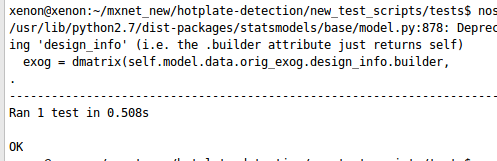Now, let’s focus on improving the value of the AUC score. Therefore, increase the milestone from 0.6 to 0.7, and start testing of the model. The results are given below -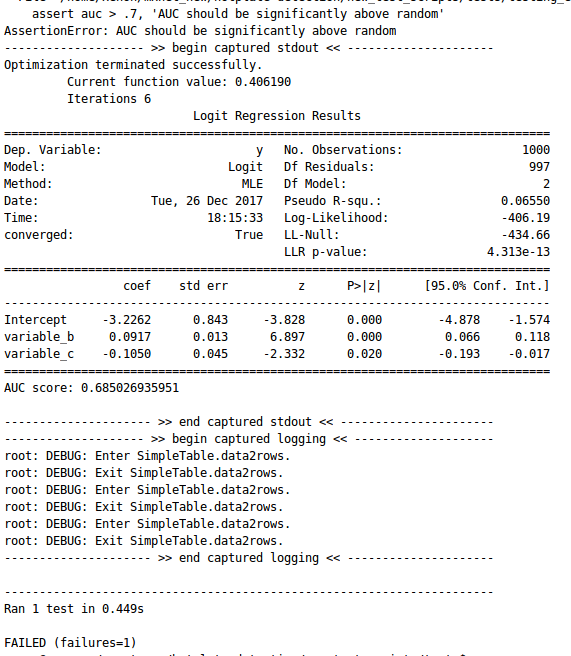After observing the above screenshot, it is noticed that the test fails due to the value of AUC. Therefore, increase the variables and test the model for variable_a, variable_b, and varibale_c. It is observed that the test is passed. The results are shown below -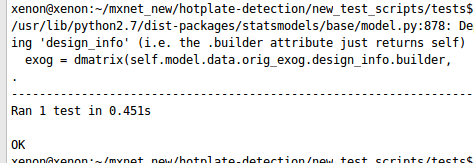Now let’s increase the milestone more to 0.79 and test again. It is observed that still, the test passed. The results are given below as screenshots -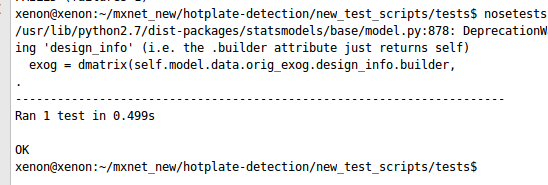The results obtained now are much better as compared with the very first model. Therefore, in this way, the accuracy of the model can be increased

### What is Backtesting?

Backtesting is the process of testing the strategies applied to a given dataset. Therefore, a Python framework named “bt” is introduced that helps to evaluate strategies and improve them. The implementation is explained below along with the code - After implementing the above code, the result is obtained which is shown below-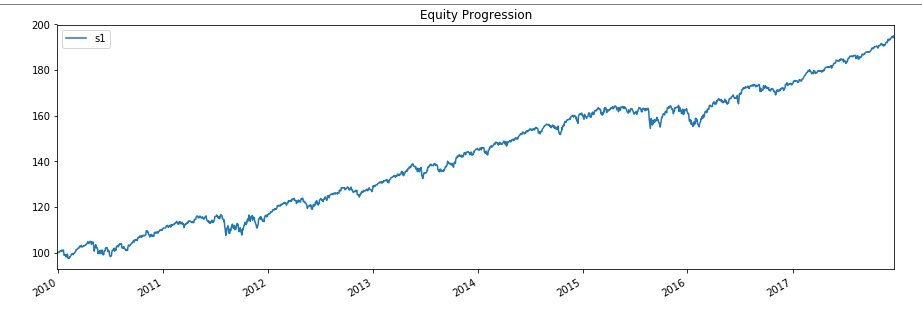Now, let’s change the strategy for the data. The code is given below - After implementing the above code, the results are obtained that are shown below -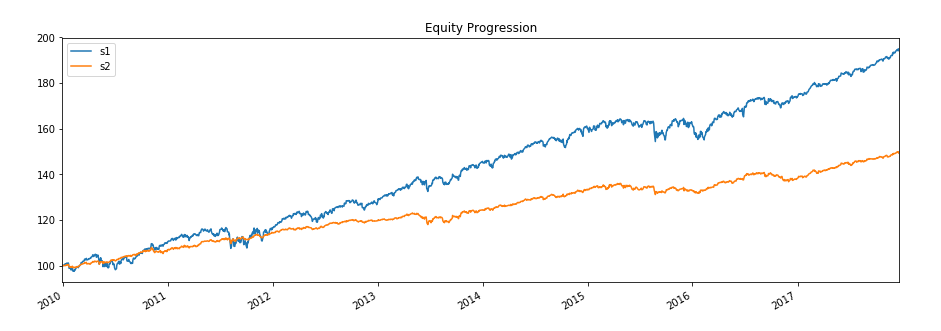The important task of this approach is to facilitate the rapid development of complex trading strategies.

## Summary of Test-Driven Machine learning and Code Coverage

Test-driven development (TDD) is a development technique where the developer must first write a test that fails before one writes a new functional code. TDD ensures a proven way to ensure effective unit testing however it does not replace traditional testing. We believe that TDD is an incredible practice that all software developers should consider during the development process.Our solutions cater to diverse industries with a focus on serving ever-changing marketing needs. Click to explore our ML Services for Productionizing Models

XenonStack follows the Test-Driven Machine learning Approach in the development of Machine learning and AI Applications with Agile Scrum Methodology.

### Text, Predictive and Statistical Modeling & Analytics

Our Data Science Services provides text analytics solutions to extract data from text sources for sentiment analysis, text clustering, and categorization using Natural Language Processing algorithms. Predict the Future, anticipate the change, nurture agility that boosts your bottom line. Build Predictive and Statistical Models for Data Products.

### WorkBench For Deep Learning Services & Machine Learning Services

At XenonStack we offer Data Science Solutions for Data scientists and researchers to Build, Deploy Machine learning and Deep Learning algorithms at scale with automated On-premises and Hybrid Cloud Solutions.

### Decision Science Solutions

XenonStack Data Visualization & Artificial Intelligence Services enables you to develop Interactive Dashboard, Data Products in form of Chat Bots, Digital Agents, and Decision Science framework, recommendation systems with progressive Web Applications Using Angular.JS, React.JS, and Reactive Programming – Scala, Go.

## Related Articles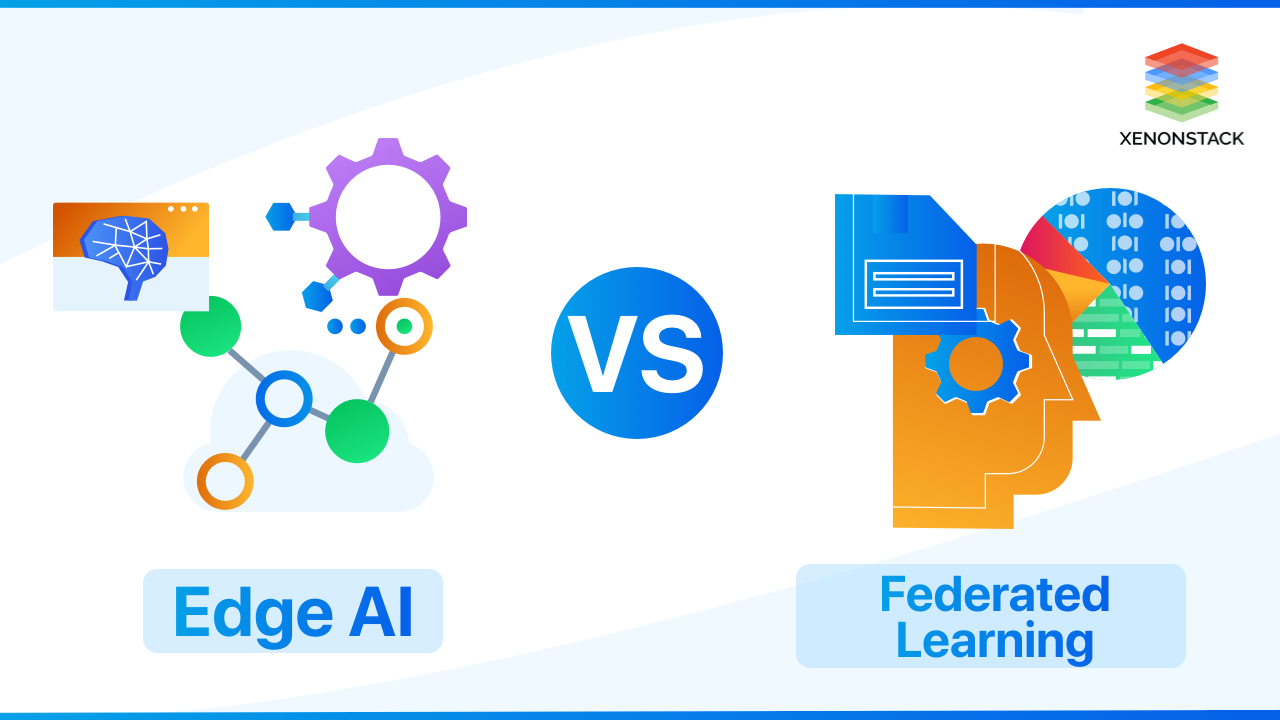Data Science

### Edge AI vs Federated Learning | Complete Overview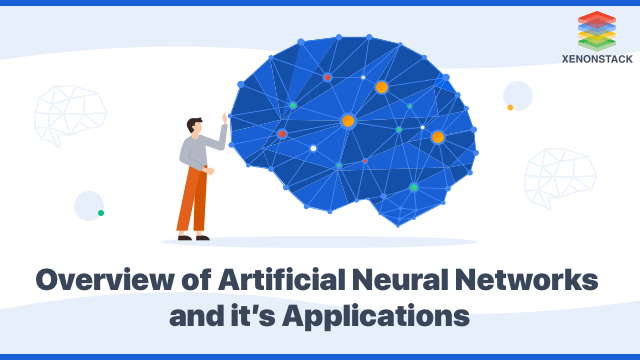Data Science

### Artificial Neural Networks Applications and AlgorithmsData Science